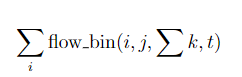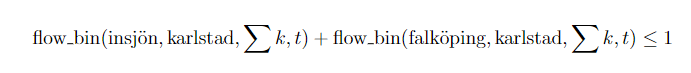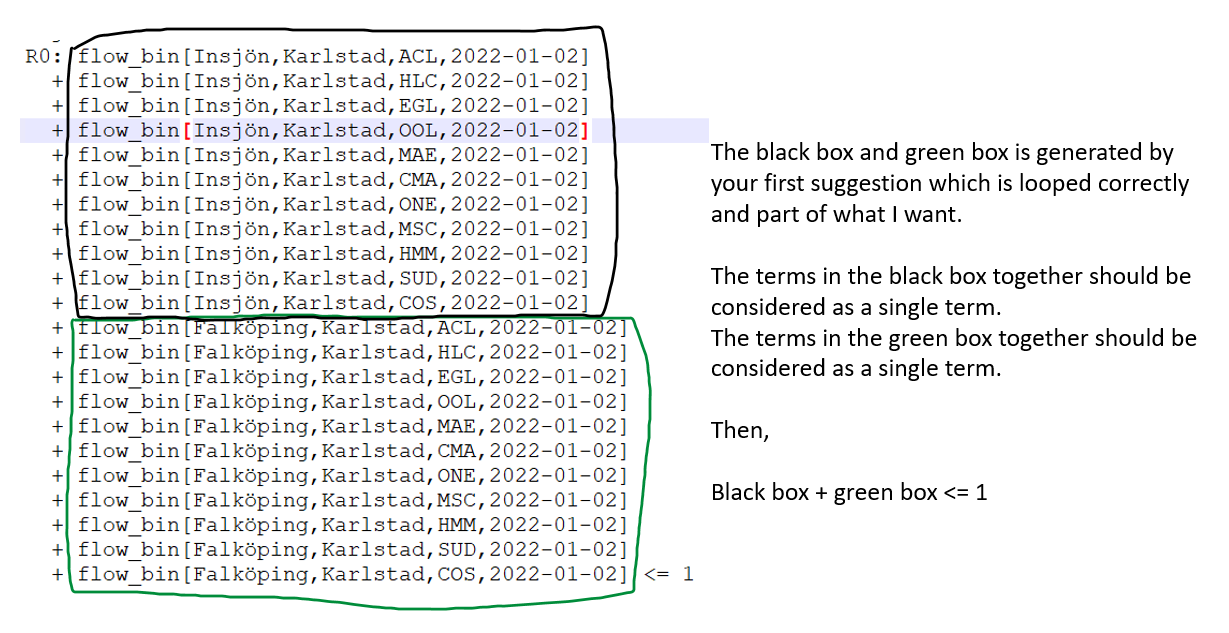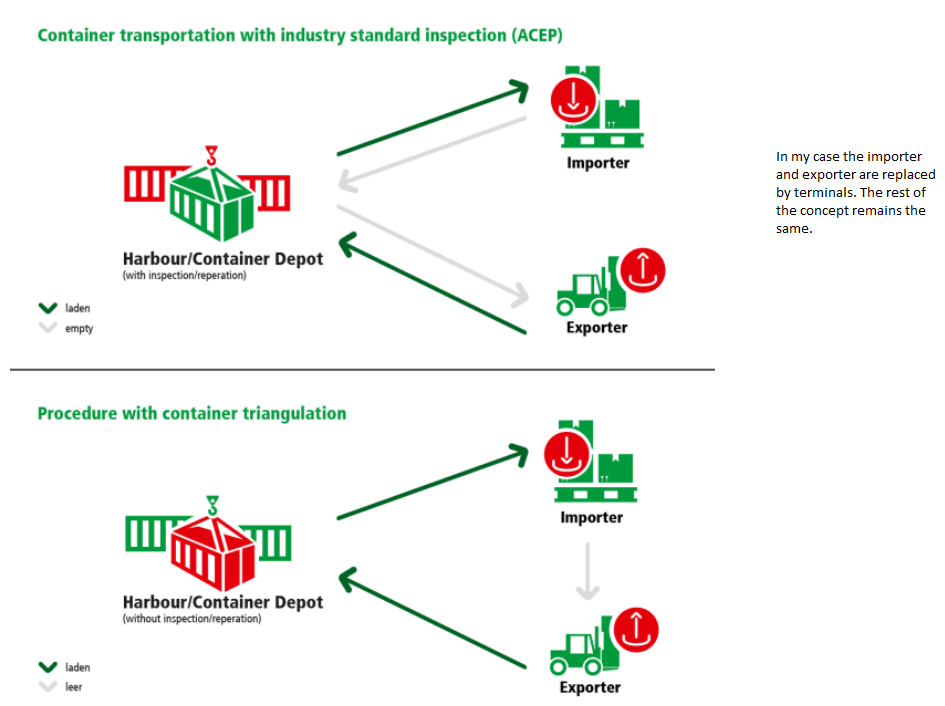•Gurobi Staff

You can pass an asterisk ($$\texttt{"*"}$$) as an argument to tupledict.sum() to sum over all values for the corresponding index. For example:

flow_bin.sum("Insjön", 'Karlstad', "*", "2022-01-02")

results in the following summation:

  flow_bin[Insjön,Karlstad,ONE,2022-01-02]+ flow_bin[Insjön,Karlstad,SUD,2022-01-02]+ flow_bin[Insjön,Karlstad,HMM,2022-01-02]+ flow_bin[Insjön,Karlstad,MAE,2022-01-02]+ flow_bin[Insjön,Karlstad,OOL,2022-01-02]+ flow_bin[Insjön,Karlstad,CMA,2022-01-02]+ flow_bin[Insjön,Karlstad,ACL,2022-01-02]+ flow_bin[Insjön,Karlstad,EGL,2022-01-02]+ flow_bin[Insjön,Karlstad,MSC,2022-01-02]+ flow_bin[Insjön,Karlstad,COS,2022-01-02]+ flow_bin[Insjön,Karlstad,HLC,2022-01-02]

Do you want a separate constraint for every $$(j, t)$$ pair? If so, try the following:

for j in inland_terminal:    for t in time:        m.addConstr(flow_bin.sum("*", j, "*", t) <= 1)

•Hej Eli,

Thanks for the input, unfortunately, I have already tried that. The output from both suggestions is not what I am looking for. To provide more context, the elements in the tuple are as follows in the same order:

i : origin

j: destination

k: operator

t: time

My requirement is to have the sum of all operators k going to a single destination from all the origins i.

Maybe the image I inserted wasn't easy to understand because of the lack of explanation.Something of this type, so the result you typed in your comment above should altogether be considered as a single term, and then the same for the next element in i. I hope the image below helps get a clearer idea.Also, all of these looped over all of the elements in time t

•Gurobi Staff

Also, all of these looped over all of the elements in time t.

Could you clarify what this means? Do you want one constraint for every combination of destination and time? That is, for each time period, each destination receives no more than one unit of flow from any origin and operator combination:

\begin{align*}\sum_i \sum_k \textrm{flow_bin}_{ijkt} &\leq 1 \qquad \forall j, t.\end{align*}

These constraints can be added with the Python code from above:

for j in inland_terminal:    for t in time:        m.addConstr(flow_bin.sum("*", j, "*", t) <= 1)

Or, do you want one constraint for every destination, and the time is included in the summation? That is, each destination receives no more than one unit of flow across all combinations of origins, operators, and times:

\begin{align*}\sum_i \sum_k \sum_t \textrm{flow_bin}_{ijkt} &\leq 1 \qquad \forall j.\end{align*}

These constraints can be added as follows:

for j in inland_terminal:    m.addConstr(flow_bin.sum("*", j, "*", "*") <= 1)
•Hej Eli,

If you see my original post with the black brackets. I want the sum of all of those terms in the first bracket to be considered as one single term and then all the terms in the second bracket to be considered as one which will be the second term. So basically j stays constant and t stays constant, then for one element in i all the elements in k are summed. Once all elements in k are summed, then we move on to the next  for the same and t  and sum of all k.

These constraints can be added with the Python code from above:

The mathematical notation is what I need but I want it to sum over all the elements in k first and only then move to iOr, do you want one constraint for every destination, and the time is included in the summation? That is, each destination receives no more than one unit of flow across all combinations of origins, operators, and times:

I want one constraint for every destination and time (time not included in summation), and each destination j should receive goods of all different operators k from only one origin i. It should not send goods of "ACL", "HMM" from i1 and goods of "MAE", "MSC" from i2.

I hope this explains it somewhat better. Thank you.

•Gurobi Staff

The mathematical notation is what I need but I want it to sum over all the elements in k first and only then move to i.

Why does the summation order matter? The result would always be the same. I.e., for a fixed $$j$$ and $$t$$:

\begin{align*}\sum_i \bigg( \sum_k \textrm{flow_bin}_{ijkt} \bigg) = \sum_i \sum_k \textrm{flow_bin}_{ijkt} &= \sum_k \sum_i \textrm{flow_bin}_{ijkt}.\end{align*}

The terms in the black box together should be considered as a single term. The terms in the black box together should be considered as a single term.

What do you hope to achieve by "grouping" together terms in a constraint? There isn't a way to define groups of terms like this in a constraint; the summation remains the same regardless of the ordering/grouping of the individual terms.

•Hej Eli,

The order of summation is not of concern but rather the grouping of terms as you mention. It's important because I cannot have incoming flows from different origins i, for different operators k. So I need all the k being sent from only one i.

If we do not group it then it will only allow one flow consisting of only one k.

The image below is what I am trying to simulate. So the operators are for containers and all containers if available should be sent from one origin i.•Gurobi Staff

So for each destination $$j$$ and time $$t$$, inbound flow for all operators must come from the same origin $$i$$? We can model this by introducing auxiliary binary variables $$z_{ij}$$ for each origin-destination pair $$(i, j)$$. The variable $$z_{ij}$$ equals $$1$$ if and only if origin $$i$$ is the designated origin for destination $$j$$.

To start, each destination should be assigned exactly one origin:

\begin{align*} \sum_i z_{ij} &= 1 \quad \forall j.\end{align*}

Next, for each destination $$j$$ and time $$t$$, no flow for any operator can come from origin $$i$$ unless $$i$$ is the designated origin for $$j$$ (i.e., $$z_{ij}$$ equals $$1$$):

\begin{align*} \textrm{flow_bin}_{ijkt} &\leq z_{ij} \quad \forall i, j, k, t. \end{align*}

Altogether in Python:

# Add auxiliary binary variablesz = m.addVars(origin_terminal, inland_terminal, vtype=GRB.BINARY, name="z")# Each destination is assigned exactly one originfor j in inland_terminal:    m.addConstr(z.sum("*", j) == 1)# Flow can pass from origin i to destination j iff z[i, j] == 1for i in origin_terminal:    for j in inland_terminal:        for k in operators:            for t in times:                m.addConstr(flow_bin[i, j, k, t] <= z[i, j])

Does this accomplish what you're trying to do? Hopefully it at least gives you some modeling ideas.

I'm assuming for each destination, the designated origin is the same for all times $$t$$. If that's not the case, you can extend this idea by adding the $$t$$ index to the auxiliary variables (i.e., $$z_{ijt}$$) and modifying the first constraint family so there's a constraint for every $$(j, t)$$ pair.